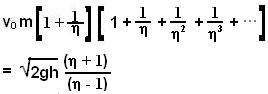## Tuesday, May 13, 2008

### Irodov Solution 1.158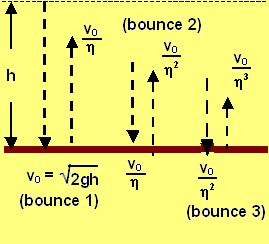The first time the ball hits the surface, it has fallen a height h i.e. lost a potential energy of mgh. This potential energy is converted into its kinetic energy and so if it hit the surface with a speed v0, then,After hitting the surface, its upwards velocity will reduce to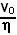. So when the ball returns to the surface to bounce a second time it impacts the surface with a speed. This time when the ball rises up it will loose a fraction of its impact velocity and bounce up with a speed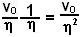. This process will continue with each bounce. In other words the ball will loose its impact velocity in a geometric progression with each bounce following the sequence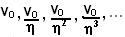.
At the kth bounce the ball impacts the surface at a speedand then reflects back with a speed. During this bounce the momentum of the ball changes fromto(the minus sign comes because the direction of velocity has reversed to up after the bounce, we have chosen the positive direction as downwards). This change is momentum is what the surface absorbs from the impact of the ball. So at the kth bounce, the ball imparts a momentum ofThe total momentum imparted by the ball it the sum of the momenta imparted after each bounce and is given by the summation of the geometric series,